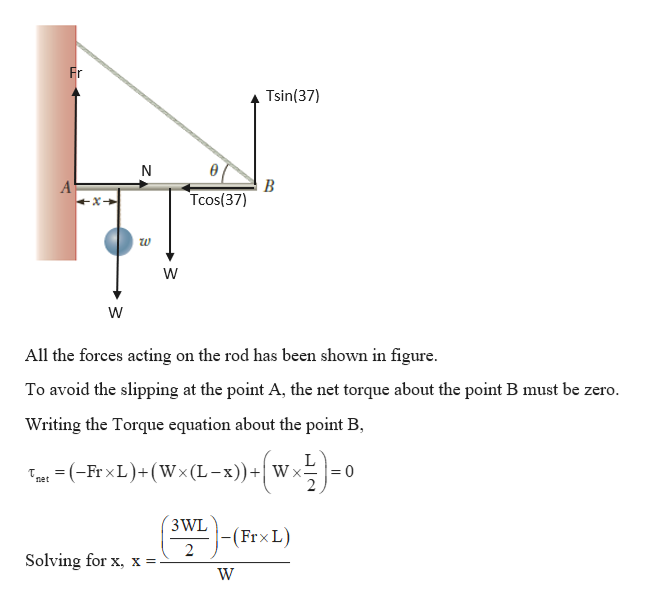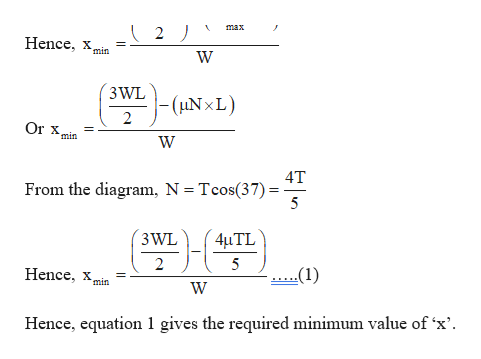Question
13 views

One end of a uniform
4.0-m-long rod of weight
w is supported by a cable
at an angle of θ = 37° with
the rod. The other end
rests against a wall, where it
is held by friction. (See Fig.
P8.36.) The coefficient of
static friction between the
wall and the rod is μs = 0.50.
Determine the minimum
distance x from point A at
which an additional weight w (the same as the weight of the
rod) can be hung without causing the rod to slip at point A.

check_circle

Step 1

Given:

μs = 0.50

Length of the rod, L = 4m

Step 2

Calculating the minimum distance ‘x’ such that the weight ‘w’ can be hung from the rod without slip:help_outlineImage TranscriptioncloseFr Tsin(37) N B Tcos(37) All the forces acting on the rod has been shown in figure. To avoid the slipping at the point A, the net torque about the point B must be zero. Writing the Torque equation about the point B, Tpet = (-Fr xL)+(Wx(L-x))+| Wx 3 WL (Fr×L) Solving for x, x =- fullscreen
Step 3

To calculate a minimum value of ‘x’, we ...help_outlineImage Transcriptionclosemax Hence, x. *min 3 WL – (HN×L) Or x min 4T From the diagram, N = Tcos(37) = 5 3 WL 4µTL 2 Hence, x -(1) min Hence, equation 1 gives the required minimum value of 'x'. fullscreen

### Want to see the full answer?

See Solution

#### Want to see this answer and more?

Solutions are written by subject experts who are available 24/7. Questions are typically answered within 1 hour.*

See Solution
*Response times may vary by subject and question.
Tagged in
SciencePhysics

### Angular Motion